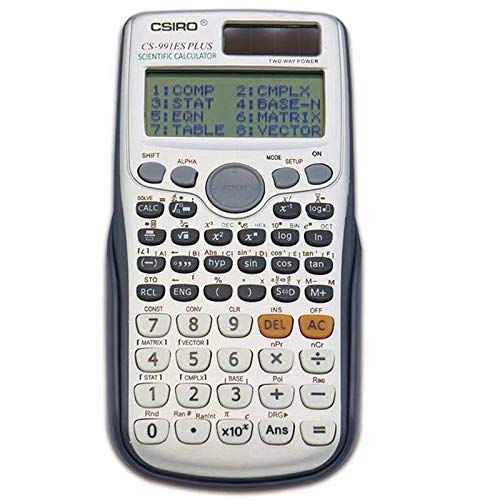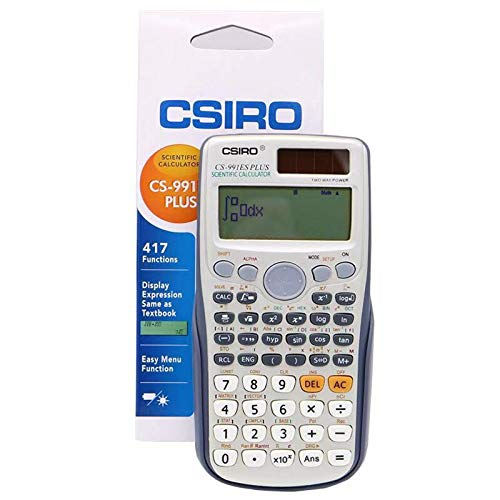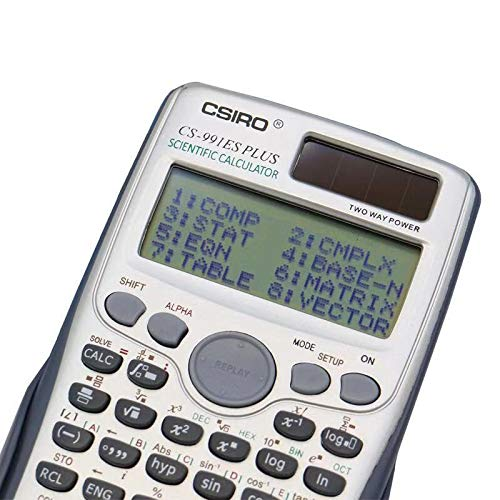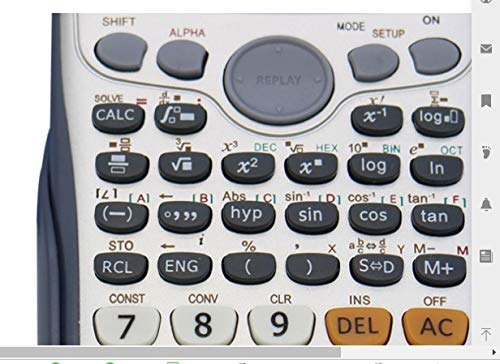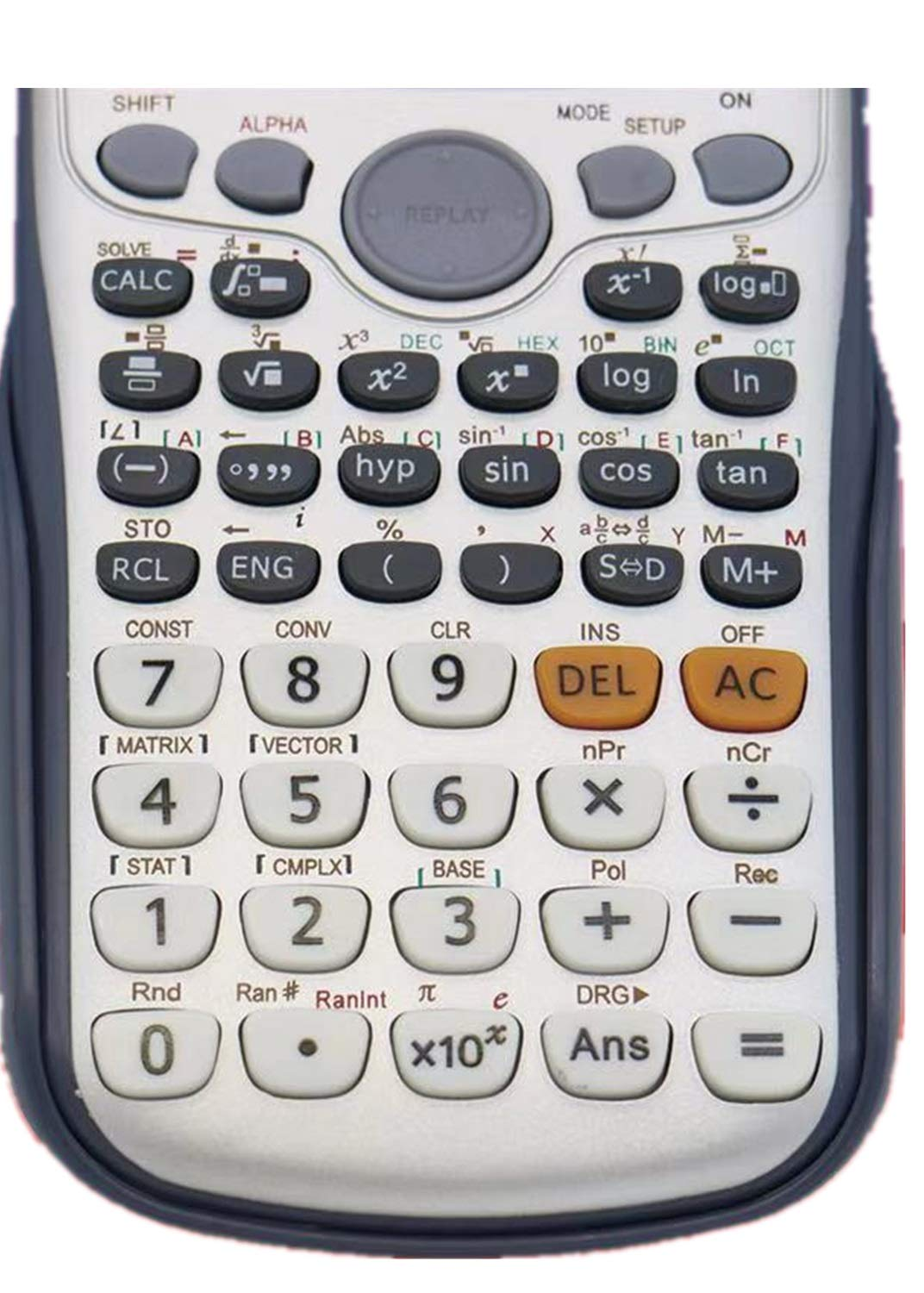# CSIRO CS-991ES Plus Engineering/Scientific Calculator

: CSIRO CS-991ES Plus Engineering/Scientific Calculator : Electronics. Natural textbook display shows expressions and results exactly as they appear in the textbook 。 417 functions, including fractions, statistics, complex number calculations, base arithmetic, linear regression, standard deviation, computer science, and polar-rectangular conversions 。 Multi-replay function allows you to backtrack step-by-step through calculations, where you can edit expressions and recalculate answers 。 Easy menu function 。 Hot ing CS-99ES PLUS Engineering/Scientific Calculator, 47 Functions, Easy Menu Function 。 。 。